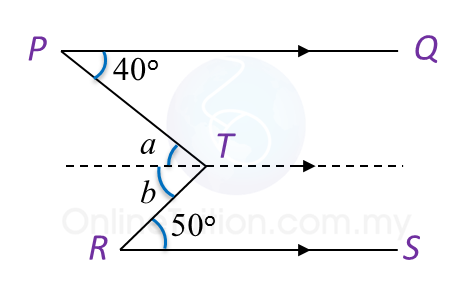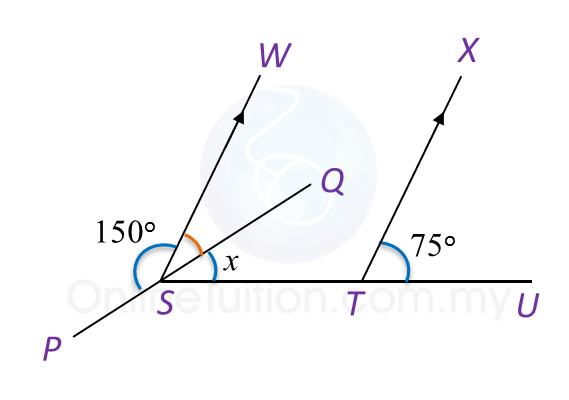# 8.1.3 Lines and Angles (Sample Questions)

Example 1:
In Diagram below,PQ is parallel to RS. Determine the value of y.Solution:Construct a line parallel to PQ and passing through W.
= 40o and b = 50o← (Alternate angles)
= a + b
= 40o+ 50o
= 90o

Example 2:
In Diagram below, PSQ and STU are straight lines. Find the value of x.Solution:
$\begin{array}{l}\angle WSQ={180}^{o}-{150}^{o}\\ \text{}={30}^{o}←\overline{)\text{Supplementary angle}}\\ \angle XTU=\angle WSQ+\angle x←\overline{)\text{Corresponding angle}}\\ \text{}{75}^{o}={30}^{o}+\angle x\\ \text{}\angle x={75}^{o}-{30}^{o}\\ \text{}\angle x={45}^{o}\\ \text{}x=45\end{array}$